## Recommended Posts

Howdy, a quick question for any math buffs out there, I am sure there is a simple answer!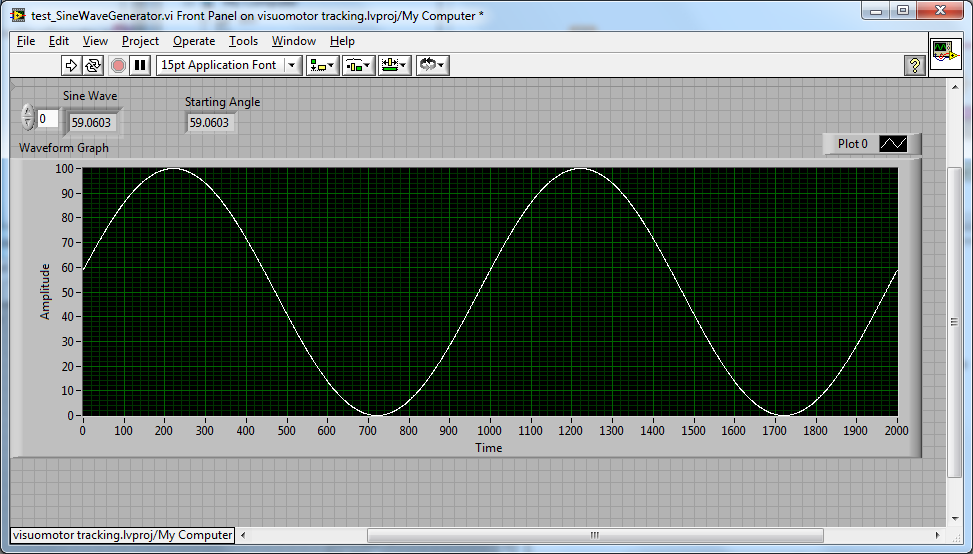I want to generate the Sine Wave as above using the following function: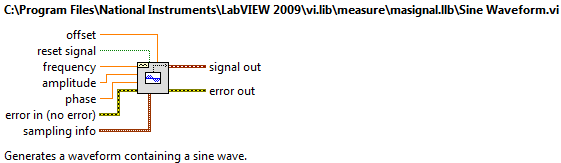My question comes from the phase input on the function - what is a formula for converting a starting angle (e.g. 60 deg) into a phase (as it is obviously non-linear)?

Also another input is Direction? I want to force which direction the curve will go from the Starting Angle (e.g. Up or Down), this will also change the phase.

Obviously I have a workaround here (as per the above graph) which is to use the function with phase set to 0 then iterate through the points and take a subset, but I am certain there is a more elegant solution... but its Saturday Night here and my brain is fried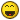Cheers

-JG

##### Share on other sites

I'm not sure what you mean by phase, but in my book that's exactly the same as the starting angle.

If you have for instance a starting angle of 90 degress, you will get a cosine wave instead of a sine.

Ton

##### Share on other sites

I'm with Ton on this one

Your example screen shot is actually starting at an "amplitude" of 60, not 60°. 90° would start at the peak of the waveform (cosine) so to start at 60° it would be much further up.

For the other question. Just use the "change sign" function on the data and start at 180-degrees or -degrees depending on that your trying to achieve..

Edited by ShaunR

##### Share on other sites

Phase of a sine wave wraps every 360 degrees, or 2*PI radian.

Some markers:

You'll get the initial slope of the sine wave to be negative for any values in the interval ]90, 270[ and positive in the intervals ]0, 90[ & ]270, 360[.

90 and 270 degrees will give an initial slope of zero (cosine and -cosine).

0 and 180 degrees will give an initial slope of 1*amplitude (sine and -sine)

Some theory:

The sine wave you're generating is of the form:

y(t) = A * sin(w*t+ phi) + offset

where

A is the maximum amplitude

w is the angular frequency and is also equal to 2*PI*frequency.

If I understand your question correctly, you'd like to be able to calculate the phase necessary to generate a wave that starts at an amplitude of 60 and specify the initial slope.

You want an initial amplitude of 60, so you know that y(t=0) = 60 and your max amplitude is 100. The equation is simple at t=0 => 60 = 100*sin(phi) or Phi = arcsin(60/100) = 0.6435 rad

To get it in degrees, divide by PI and multiply by 180. ==> 36.87 deg

Your solution will be slightly changed if your offset is not zero...

Sine Phase.vi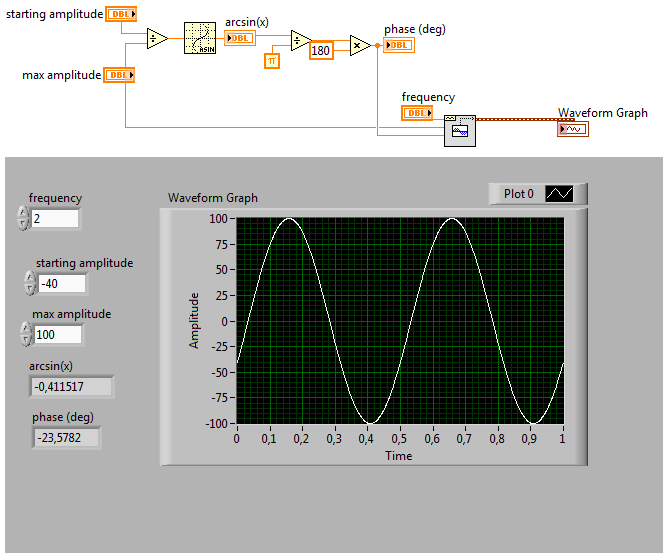•1

##### Share on other sites

y(t) = A * sin(w*t+ phi) + offset

Sorry if there was confusion in my question, but François nailed what I was after, cheers!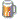Here is the change accounting for an offset (e.g. 90-160 deg waveform) if anyone is interested.

This solution is much nicer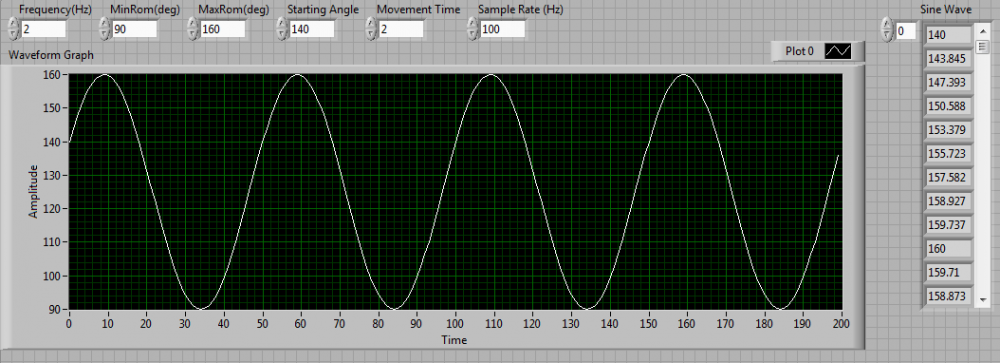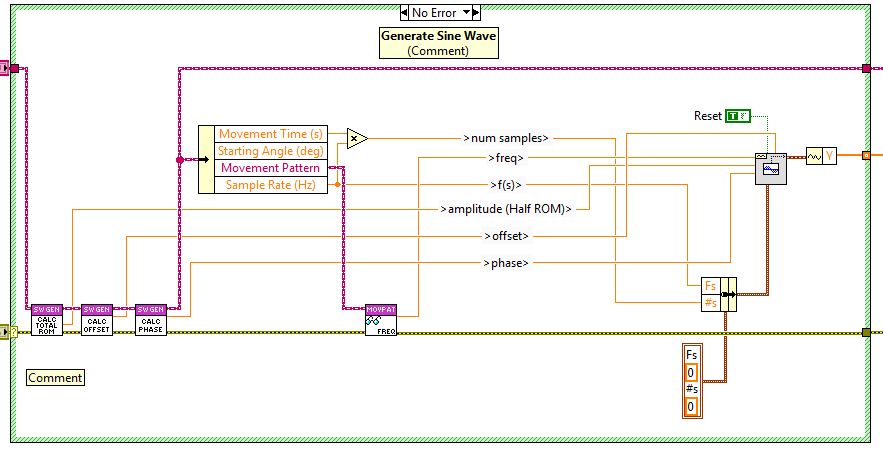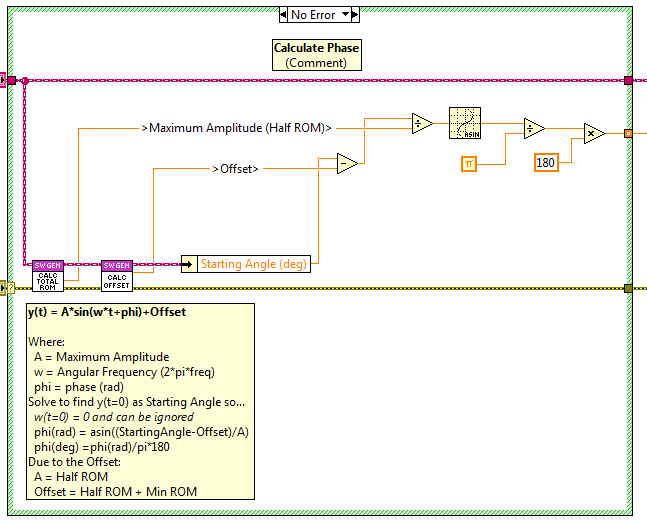## Join the conversation

You can post now and register later. If you have an account, sign in now to post with your account.×   Pasted as rich text.   Paste as plain text instead

Only 75 emoji are allowed.

×   Your previous content has been restored.   Clear editor

×   You cannot paste images directly. Upload or insert images from URL.

×

×